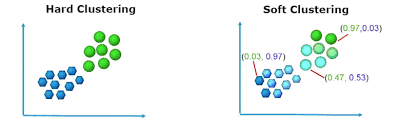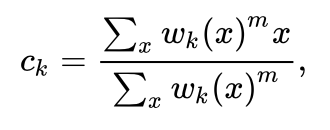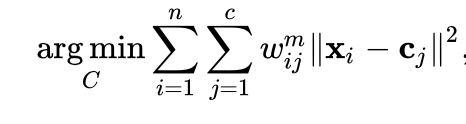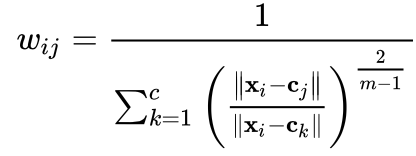# Fuzzy Clustering

## Introduction

Fuzzy clustering is a common buzzword when we talk about clustering in unsupervised learn and it’s claimed to be better than the trivial clustering algorithm. Now the question might arise in your mind, what makes fuzzy clustering different from the normal clustering algorithm? Let’s try to answer this question in this blog.## What is clustering?

Clustering is a very powerful unsupervised machine learning technique that divides the data points into clusters based on how similar the data points are with each other. The clustering algorithm in unsupervised machine learning is segregated into two categories:

### Hard Clustering

In hard clustering we talk about whether a particular data point belongs to a cluster or not. In other words, we can say that each datapoint is assigned only a single cluster. The K-Means, K-Medians clustering algorithms are hard clustering algorithms. To read more about these algorithms refer here.

### Soft Clustering

On the other hand in soft clustering each data point belongs to a cluster with a certain probability or likelihood, i.e., each data point has its own membership of a cluster. FCM(Fuzzy C-means clustering) algorithm is an example of soft clustering.## What is Fuzzy Clustering?

Fuzzy clustering is an unsupervised machine learning algorithm which comes under the soft clustering category. As the name suggests, i.e., it creates fuzzy clusters. There is no clear decision to which cluster a data point belongs. Rather it uses the concept of likelihood of membership of data point to a particular cluster. We can say that a data point has a greater membership in a cluster if it’s nearer to it. Let’s look at some terminologies used in fuzzy clustering.

## Terminologies used in Fuzzy Clustering

### Degrees of fuzziness(m)

The value of m determines the extent to which a particular cluster is fuzzy. It is a real number which lies in the range from 1.0 to infinity. So  closer is the value of m to 1.0, the similarity between the trivial hard clustering algorithm and fuzzy clustering would increase.

### Centroid of the kth cluster(ck)

This is a common term used to denote the centroid of a cluster which is given byHere wk(x) is the weight associated with a point x to be a part of kth cluster and m is the degree of fuzziness.

### Partition/Weight Matrix(W)

The partition matrix W contains the weights/degree of a particular data-point belonging to a part of a cluster.

## Algorithm for Fuzzy Clustering

Let X = {X1, X2, ….., Xn} be a set of points to be assigned in different clusters. Let c be the number of cluster centers, i.e., C = {C1, C2, ….., Cc}. Let W be a partition/weight matrix where W = Wi, j which lies in the range 0 to 1 and is read as the weight of the ith data point belonging to the jth cluster.

Step1: Choose a number c as the number of clusters.

Step2: Assign the weights randomly to each data point by randomly initialising the matrix.

Step3: Repeat the following steps until the convergence is achieved:

1. Calculate the centers in each iteration.
2. For each data point compute the membership in each cluster and update the weight matrix.

In each step we will be optimizing the objective function which is similar to the k-means clustering algorithmThe weights will be updated using the following formula.NOTE: refer to the terminologies for understanding what are the terms used in the above formulae.

## Applications of Fuzzy Clustering

Image Analysis

It’s used to improve the efficiency of clustering under noise which is a typical challenge in image processing. It’s also to differentiate between different activities in an image.

BioInformatics

It’s used in pattern recognition techniques which is very helpful while analysing gene sequences. Hence it allows us to look at the sequences to which a particular pattern belongs.

1. What is fuzzy clustering?
It is an unsupervised machine learning algorithm which falls under soft clustering where each data point has a membership associated with each cluster unlike the standard k-means clustering algorithm.

2. Are there any further improvisations in the fuzzy algorithm?
Yes, there have been many improvements in the fuzzy clustering algorithm like the fuzzy c-means++ algorithm, also there have been many improvisations by introducing regularisation terms.

## Key takeaways

This article gave a brief introduction to what is fuzzy clustering. We saw different types of clustering and in which category does fuzzy clustering fall in. Also we looked at various terminologies and algorithms of Fuzzy clustering along with its pros and cons. To dive deeper into machine learning, check out our industry-level courses on coding ninjas.